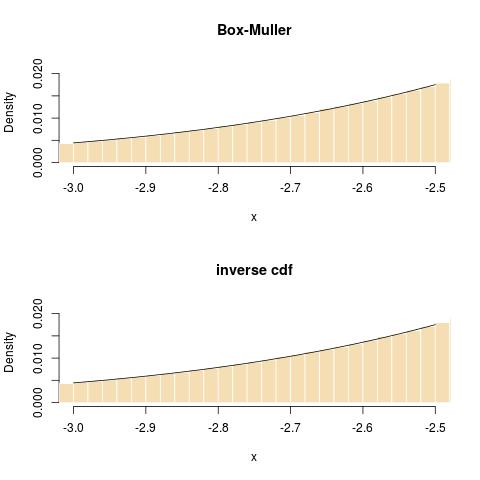on approximations of Φ and Φ⁻¹

Posted in Books, Kids, R, Statistics with tags , , , , , , , , , on June 3, 2021 by xi'an

As I was working on a research project with graduate students, I became interested in fast and not necessarily very accurate approximations to the normal cdf Φ and its inverse. Reading through this 2010 paper of Richards et al., using for instance Polya’s$F_0(x) =\frac{1}{2}(1+\sqrt{1-\exp(-2x^2/\pi)})$

(with another version replacing 2/π with the squared root of π/8) and$F_2(x)=1/1+\exp(-1.5976x(1+0.04417x^2))$

not to mention a rational faction. All of which are more efficient (in R), if barely, than the resident pnorm() function.

test replications elapsed relative user.self
3 logistic       100000   0.410    1.000     0.410
2    polya       100000   0.411    1.002     0.411
1 resident       100000   0.455    1.110     0.455

For the inverse cdf, the approximations there are involving numerical inversion except for$F_0^{-1}(p) =(-\pi/2 \log[1-(2p-1)^2])^{\frac{1}{2}}$

which proves slightly faster than qnorm()

test replications elapsed relative user.self
2 inv-polya       100000   0.401    1.000     0.401
1  resident       100000   0.450    1.000     0.450

simulation by inverse cdf

Posted in Books, Kids, R, Statistics, University life with tags , , , , , on January 14, 2015 by xi'an

Another Cross Validated forum question that led me to an interesting (?) reconsideration of certitudes! When simulating from a normal distribution, is Box-Muller algorithm better or worse than using the inverse cdf transform? My first reaction was to state that Box-Muller was exact while the inverse cdf relied on the coding of the inverse cdf, like qnorm() in R. Upon reflection and commenting by other members of the forum, like William Huber, I came to moderate this perspective since Box-Muller also relies on transcendental functions like sin and log, hence writing$X=\sqrt{-2\log(U_1)}\,\sin(2\pi U_2)$

also involves approximating in the coding of those functions. While it is feasible to avoid the call to trigonometric functions (see, e.g., Algorithm A.8 in our book), the call to the logarithm seems inescapable. So it ends up with the issue of which of the two functions is better coded, both in terms of speed and precision. Surprisingly, when coding in R, the inverse cdf may be the winner: here is the comparison I ran at the time I wrote my comments

> system.time(qnorm(runif(10^8)))
sutilisateur     système      écoulé
10.137           0.120      10.251
> system.time(rnorm(10^8))
utilisateur     système      écoulé
13.417           0.060      13.472`

However re-rerunning it today, I get opposite results (pardon my French, I failed to turn the messages to English):

> system.time(qnorm(runif(10^8)))
utilisateur     système      écoulé
10.137       0.144      10.274
> system.time(rnorm(10^8))
utilisateur     système      écoulé
7.894       0.060       7.948

(There is coherence in the system time, which shows rnorm as twice as fast as the call to qnorm.) In terms, of precision, I could not spot a divergence from normality, either through a ks.test over 10⁸ simulations or in checking the tails:“Only the inversion method is inadmissible because it is slower and less space efficient than all of the other methods, the table methods excepted”. Luc Devroye, Non-uniform random variate generation, 1985

Update: As pointed out by Radford Neal in his comment, the above comparison is meaningless because the function rnorm() is by default based on the inversion of qnorm()! As indicated by Alexander Blocker in another comment, to use an other generator requires calling RNG as in

RNGkind(normal.kind = “Box-Muller”)

(And thanks to Jean-Louis Foulley for salvaging this quote from Luc Devroye, which does not appear to apply to the current coding of the Gaussian inverse cdf.)